### Home > A2C > Chapter Ch11 > Lesson 11.2.3 > Problem11-71

11-71.
1. Solve each of the following equations. Homework Help ✎

1.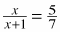2.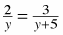3.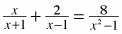4.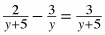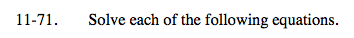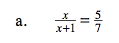7x = 5x + 5

$x=\frac{5}{2}$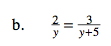See part (a).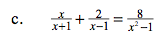Multiply by (x + 1)(x − 1).

(x − 1)x + 2(x + 1) = 8

x = −3, x = 2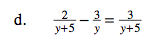See part (a).